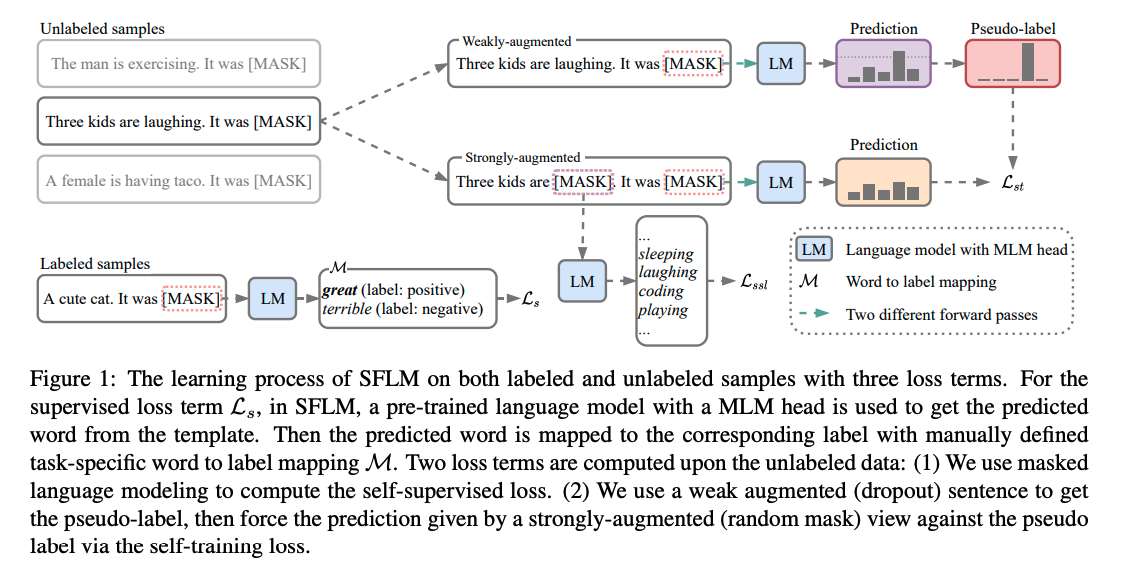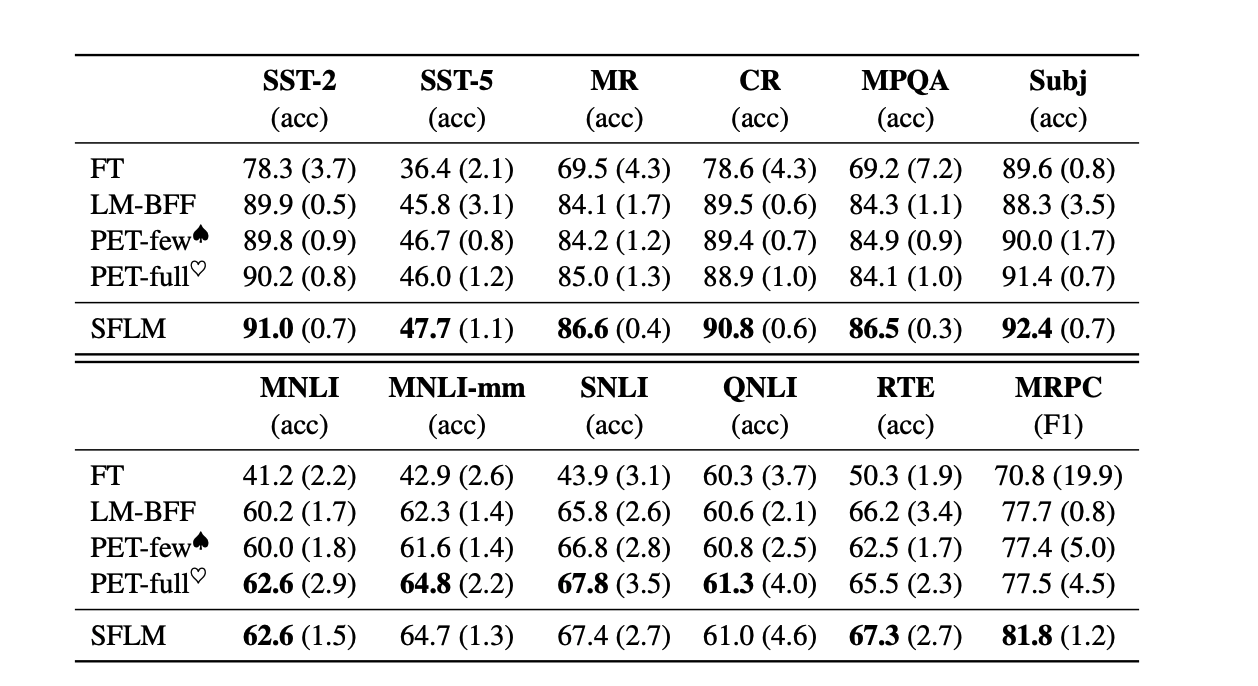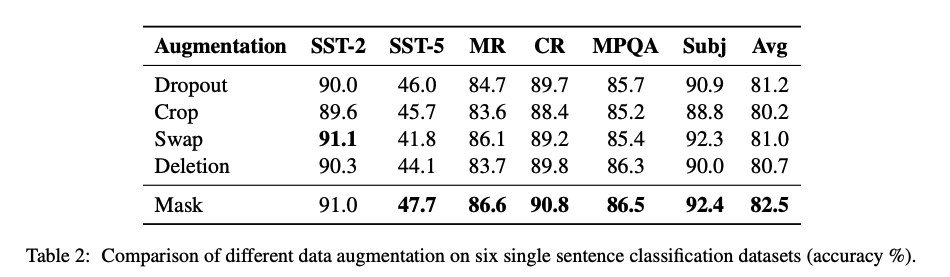## 方法SFLM训练过程包含两部分：有监督训练和无监督训练。对于有标注数据，进行常规的prompt模式有监督训练过程即可，这里我们主要重点介绍无监督训练过程，从上图可以看到，对于每个未标注的样本（prompt形式），使用弱增强和强增强获得对应增强样本。首先，弱增强样本通过模型得到预测得分，当模型的预测得分高于一定的阈值时，对应的类别作为 ground truth 的标签，即伪标签。然后，强增强的样本通过相同模型得到预测得分，并使用交叉熵损失将此预测得分与 ground truth 伪标签进行比较。

SFLM损失包含三部分：有标签的样本用有监督的损失$L_s$，没有标签的样本包含自训练的损失$L_{st}$和自监督的损失$L_{ssl}$， 三个损失都是标准的交叉熵损失。

Prompt-based supervised loss

$p_{m}\left(y_{i} \mid x_{i}\right)=p_{m}\left([\text { MASK }]=\mathcal{M}^{\prime}\left(y_{i} \mid x_{i}^{\text {prompt }}\right)\right.$

$x_{i}^{p r o m p t}=x_{i} \circ \text { It was [MASK] }$

$\mathcal{L}_{s}=\frac{1}{B} \sum_{i=1}^{B} H\left(y_{i}, p_{m}\left(y_{i} \mid x_{i}\right)\right)$

Self-training loss

\begin{aligned} \mathcal{L}_{s t}=& \frac{1}{\mu B} \sum_{i=1}^{\mu B} \mathbb{1}\left(\max \left(q_{i}\right) \geq \tau\right) \\ & \mathrm{H}\left(\hat{q}_{i}, p_{m}\left(y_{i} \mid \mathcal{A}\left(u_{i}\right)\right)\right) \end{aligned}

• 弱增强: 采用dropout方式，弱监督仍然保持原始句子语义。

Self-supervised loss

$\mathcal{L}=\mathcal{L}_{s}+\lambda_{1} \mathcal{L}_{s t}+\lambda_{2} \mathcal{L}_{s s l}$

## 实验

### 实验结果